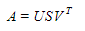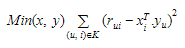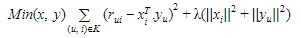1648700310

# Mathematical Overview | The Singular Value Decomposition (SVD)

This video presents a mathematical overview of the singular value decomposition (SVD).

Singular Value Decomposition (SVD) is a widely used technique to decompose a matrix into several component matrices, exposing many of the useful and interesting properties of the original matrix. Using SVD, we can determine the rank of the matrix, quantify the sensitivity of a linear system to numerical error, or obtain an optimal lower-rank approximation to the matrix.

These lectures follow Chapter 1 from:  "Data-Driven Science and Engineering: Machine Learning, Dynamical Systems, and Control"  by Brunton and Kutz

Book Website: http://databookuw.com

Book PDF: http://databookuw.com/databook.pdf

Brunton Website: http://eigensteve.com

## Buddha Community1597123980

## Recommender System — singular value decomposition (SVD) & truncated SVD

The most common method for recommendation systems often comes with Collaborating Filtering (CF) where it relies on the past user and item dataset. Two popular approaches of CF are latent factor models, which extract features from user and item matrices and neighborhood models, which finds similarities between products or users.

The neighborhood model is an item-oriented approach to discover the user preference based on the ratings given by the user for similar items. On the other hand, latent factor models such as Singular Value Decomposition (SVD) extract features and correlation from the user-item matrix. For example, when items are movies in different categories. SVD would generate factors when looking into the dimension space like action vs comedy, Hollywood vs Bollywood, or Marvel vs Disney. Mainly, we will focus on the latent factor model for the Singular Value Decomposition (SVD) approach.

In this article, you will learn the singular value decomposition and truncated SVD of the recommender system:

(1) Introduction to singular value decomposition

(2) Introduction to truncated SVD

(3) Hands-on experience of python code on matrix factorization

## Introduction to singular value decomposition

When it comes to dimensionality reduction, the Singular Value Decomposition (SVD) is a popular method in linear algebra for matrix factorization in machine learning. Such a method shrinks the space dimension from N-dimension to K-dimension (where K<N) and reduces the number of features. SVD constructs a matrix with the row of users and columns of items and the elements are given by the users’ ratings. Singular value decomposition decomposes a matrix into three other matrices and extracts the factors from the factorization of a high-level (user-item-rating) matrix.Matrix U: singular matrix of (user*latent factors)

Matrix S: diagonal matrix (shows the strength of each latent factor)

Matrix U: singular matrix of (item*latent factors)

From matrix factorization, the latent factors show the characteristics of the items. Finally, the utility matrix A is produced with shape m*n. The final output of the matrix A reduces the dimension through latent factors’ extraction. From the matrix A, it shows the relationships between users and items by mapping the user and item into r-dimensional latent space. Vector X_i is considered each item and vector Y_u is regarded as each user. The rating is given by a user on an item as R_ui = X^T_i * Y_u. The loss can be minimized by the square error difference between the product of R_ui and the expected rating.Regularization is used to avoid overfitting and generalize the dataset by adding the penalty.#recommendation-system #matrix #singular-values #machine-learning #python1648138512

## Singular Value Decomposition (SVD) | Overview of the Singular Value Decomposition

This video presents an overview of the singular value decomposition (SVD), which is one of the most widely used algorithms for data processing, reduced-order modeling, and high-dimensional statistics.

Singular Value Decomposition (SVD) is a widely used technique to decompose a matrix into several component matrices, exposing many of the useful and interesting properties of the original matrix. Using SVD, we can determine the rank of the matrix, quantify the sensitivity of a linear system to numerical error, or obtain an optimal lower-rank approximation to the matrix.1648700310

## Mathematical Overview | The Singular Value Decomposition (SVD)

This video presents a mathematical overview of the singular value decomposition (SVD).

Singular Value Decomposition (SVD) is a widely used technique to decompose a matrix into several component matrices, exposing many of the useful and interesting properties of the original matrix. Using SVD, we can determine the rank of the matrix, quantify the sensitivity of a linear system to numerical error, or obtain an optimal lower-rank approximation to the matrix.

These lectures follow Chapter 1 from:  "Data-Driven Science and Engineering: Machine Learning, Dynamical Systems, and Control"  by Brunton and Kutz

Book Website: http://databookuw.com

Book PDF: http://databookuw.com/databook.pdf

Brunton Website: http://eigensteve.com1658204077

## Singular Value Decomposition (SVD) and Image Compression

The Singular Value Decomposition (SVD) of a matrix is a factorization of that matrix into three matrices. It has some interesting algebraic properties and conveys important geometrical and theoretical insights about linear transformations. It also has some important applications in data science.

In this video, we learn a very useful matrix trick called singular value decomposition (SVD), in which we express a matrix as a product of two rotation matrices and one scaling matrix.
We also show a very interesting application to image compression.

Introduction: (0:00)
Transformations: (0:50)
A puzzle: (1:27)
A harder puzzle: (2:21)
Linear transformations: (3:50)
Dimensionality reduction: (10:50)
Image compression: (23:57)1598662200

## The Singularity: When Will We Transition to a Dictatorship of Robots?

Technological Singularity: the moment when robots reach a level of intelligence that is superior to the current level of human reasonableness or ability to predict the outcomes of unrestrained technological growth. As a consequence, the economic, cultural and political singularity of human civilization follows. And although the singularity is still the subject of many Hollywood films and fictions, the possibility of this hypothetical moment genuinely approaching is looking more and more likely.

The process of replacing the biological by technological has already approached the substitution of a human by some algorithm. Thanks to technological acceleration and the accumulation of historical time, the desire to go beyond biology brings mankind closer to the culmination point — the singularity. Acceleration of historical time, that is, the concentration of social events, followed by mankind in a unit of time — is an important factor in the concept of singularity.

The singularity really _is _somewhere near. Moreover, its hypothetical date is real to calculation by scientists, and such data has been presented to the public. After making their calculations, scientists claim to have easily found the starting point of the “singularity”.

It occurs around the middle of the 21st century, which coincides with the forecasts by Ray Kurzweil, who, based on the dynamics of the exponential development of computer technology, predicts that:

• · By 2029 the machine will pass the Turing test;
• · By 2034 artificial intelligence will be created;
• · By the 2040s the non-biological mind will be more powerful than the biological one;
• · By the end of 2040 the Earth will turn into a reasonable “computer”, more precisely, a networked intelligent being;
• · At the end of the century this computer will cover the Universe with its powers

#artificial-intelligence #robotics #machine-learning #future #the-singularity #what-is-the-singularity-theory #hackernoon-top-story #when-will-singularity-happen